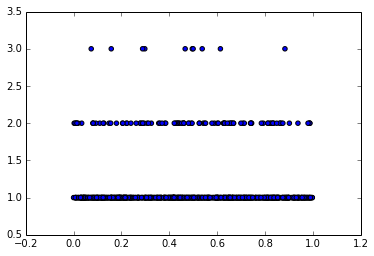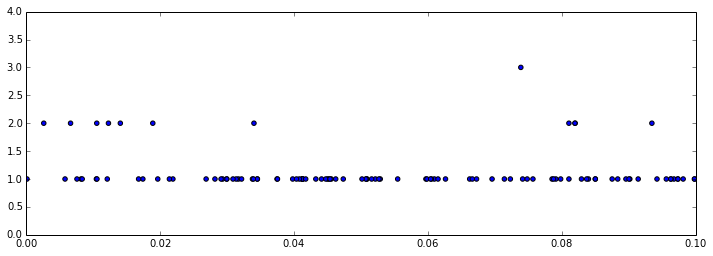# Visualization of cardinality algorithm (naive version)

In :
import uuid
import math
%matplotlib inline


Create a list of 10 random UUIDs

In :
list10 = [str(uuid.uuid4()) for i in xrange(10)]
list10

Out:
['0084d80c-283c-4392-947f-a3975ae4c88a',
'9ee0603d-f5bb-40ee-8e26-88668300cdbe',
'993c2ba5-1821-4fc8-acf6-f2349045aa5f',
'9f15bd5e-f872-4fbd-a0cb-a6b6c1e354d4',
'48285124-11c6-4060-8b93-3aec01ef6fa8',
'11e632f1-665a-4074-a0fe-8a4e3ff1b83b',
'4d1b272f-b269-4716-9418-4d7fcce650fd',
'a46cbf0d-ca3e-4dbf-8ab2-1240f5d96118',
'd3e892b8-0548-4e25-98ef-65cf80959dd7']

Now lists of 100 and 1000 UUIDs

In :
list100 = [str(uuid.uuid4()) for i in xrange(100)]
list1000 = [str(uuid.uuid4()) for i in xrange(1000)]


Hash the list of 10 UUIDs with a hash function that maps to floating point numbers in the range [0,1)

In :
h10 = [hash(x) / pow(2.0,32.0) + 0.5 for x in list10]


Find the smallest, and the exponentiate that to get the approximation of the size (cardinality) of the set

In :
m10 = min(h10)
m10

Out:
0.07389730727300048
In :
pow(10,-math.log10(m10))

Out:
13.532292811504993

Do the same for the sets of 100 and 1000

In :
h100 = [hash(x) / pow(2.0,32.0) + 0.5 for x in list100]

In :
m100 = min(h100)
m100

Out:
0.0026511941105127335
In :
pow(10,-math.log10(m100))

Out:
377.1885264963127
In :
h1000 = [hash(x) / pow(2.0,32.0) + 0.5 for x in list1000]

In :
m1000 = min(h1000)
m1000

Out:
0.00014685397036373615
In :
pow(10,-math.log10(m1000))

Out:
6809.485623869372

Plot the hashed values. Top set of dots is for the set of 10 hashed UUIDs, middle set is for the set of 100 hashed UUIDs, and the bottom set is for the set of 1000 hashed UUIDs

In :
scatter(h10 + h100 + h1000, *10 + *100 + *1000)

Out:
<matplotlib.collections.PathCollection at 0xb385860>Zoom in to the left portion of the above plot.

In :
fig, ax = plt.subplots()
fig.set_size_inches(12,4)
ax.set_xlim([0, 0.1])
ax.set_ylim([0, 4])
ax.scatter(h10 + h100 + h1000, *10 + *100 + *1000)

Out:
<matplotlib.collections.PathCollection at 0xb71e908># Improving the accuracy

We can improve the accuracy by splitting the original set into m subsets, applying the above naive algorithm over each of the m subsets, and averaging the results together.

Below we do so for the set of 1000 hashed UUIDs, where we split it up into 10 subsets of 100 each, average the resulting min hash values, exponentiate that average, and then multiply that back by 10 to get the cardinality of the whole set.

In :
betterm1000=mean([min(h1000[x:x+100]) for x in xrange(10)])

In :
10*pow(10,-math.log10(betterm1000))

Out:
358.90695792638485

As you can see, this resulted in a result that is off by a factor of 3, instead of off by a factor of 7 as before.

There are even more clever and accurate ways to average the mins, such as using a geometric average, or the ultimate, the HyperLogLog algorithm for averaging as described in HyperLogLog: the analysis of a near-optimal cardinality estimation algorithm.

In [ ]: